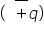# Electric Field Lines

Electric lines of force:

An electric lines of force may be defined as the curve along which a small positive charge would tend to move when free to do so in an electric field and tangent to which at any point gives the direction of the electric field at that point.

Properties of electric lines of force:

1. The lines of force are continuous smooth curve without any breaks.
2. The lines of force starts at positive charge and end at negative charges. They cannot form closed loops. If there is a single charge, then the lines of will start or end at infinity.
3. The tangent to a line of force at any point gives the direction of the electric fields at that point.
4. No two line of force can cross each other.
5. The lines of force are close together in a strong field.
6. The lines of force are far apart in a weal field.
7. The lines of force parallel and equally spaced in a uniform field.
8. The electric field inside a charged conductor is zero so the lines of force do not pass through a conductor.

Electric field lines for different charged conductors :

1.Field lines of a positive point charge: fig. shows the lines of force of an isolated positive point charge. They are directed radially outwards because a small positive charge would be accelerated in the outward direction. They extend to infinity. The field is spherically symmetric. i.e. it looks same in all directions, as seen from the point charge.

2.Field lines of a negative point charge: the electric field of a negative charge is also spherically symmetric but the lines of force points radially inward as shown in fig..  They start from infinity.

3.Field lines of two equal and opposite charges :  Fig. shows the electric lines of force of an electric dipole, i.e. a system of two equal and opposite point chargesseparated by a small distance. They start from the positive charge and end on the negative charge. The lines of force seem to contract lengthwise as if the two charges are being pulled together. The field is cylindrically symmetric about the dipole axis, i.e. the field pattern is same in all planes passing through the dipole axis. Clearly, the electric field at all points on the equatorial lines is parallel to the axis of the dipole.

4.Field lines of two equal and positive point charges : fig. shows the lines of force of two equal and positive point charges. They seen to exert a lateral pressure as if the two charges are being pushed away from each other. This explains repulsion between two like charges. The field is zero at the middle point N of the join of two charges. This point is called neutral point from which no lines of force passes. This field also has cylindrical symmetry.

5.Field lines of a positively charged plane conductor :

A small positive charge would tend to move normally away from the plane conductor. Thus the lines of force are parallel and normal to the surface of the conductor. They are equidistant, indicating that electric field E is uniform at all points near the plane conductor.

Related Keywords